# modelAccuracyPlot

Scatter plot of predicted and observed EADs

## Syntax

``modelAccuracyPlot(eadModel,data)``
``modelAccuracyPlot(___,Name,Value)``
``h = modelAccuracyPlot(ax,___,Name,Value)``

## Description

example

````modelAccuracyPlot(eadModel,data)` returns a scatter plot of observed vs. predicted exposure at default (EAD) data with a linear fit. `modelAccuracyPlot` supports comparison against a reference model. By default, `modelAccuracyPlot` plots in the EAD scale. ```

example

````modelAccuracyPlot(___,Name,Value)` specifies options using one or more name-value pair arguments in addition to the input arguments in the previous syntax. You can use the `ModelLevel` name-value pair argument to compute metrics using the underlying model's transformed scale. ```

example

````h = modelAccuracyPlot(ax,___,Name,Value)` specifies options using one or more name-value pair arguments in addition to the input arguments in the previous syntax and returns the figure handle `h`.```

## Examples

collapse all

This example shows how to use `fitEADModel` to create a `Tobit` model and then use `modelAccuracyPlot` to generate a scatter plot for predicted and observed EADs.

```load EADData.mat head(EADData)```
```ans=8×6 table UtilizationRate Age Marriage Limit Drawn EAD _______________ ___ ___________ __________ __________ __________ 0.24359 25 not married 44776 10907 44740 0.96946 44 not married 2.1405e+05 2.0751e+05 40678 0 40 married 1.6581e+05 0 1.6567e+05 0.53242 38 not married 1.7375e+05 92506 1593.5 0.2583 30 not married 26258 6782.5 54.175 0.17039 54 married 1.7357e+05 29575 576.69 0.18586 27 not married 19590 3641 998.49 0.85372 42 not married 2.0712e+05 1.7682e+05 1.6454e+05 ```
```rng('default'); NumObs = height(EADData); c = cvpartition(NumObs,'HoldOut',0.4); TrainingInd = training(c); TestInd = test(c);```

Select Model Type

Select a model type for `Tobit` or `Regression`.

`ModelType =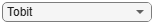"Tobit";`

Select Conversion Measure

Select a conversion measure for the EAD response values.

`ConversionMeasure =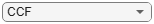"CCF";`

Create `Tobit` EAD Model

Use `fitEADModel` to create a `Tobit` model using `EADData`.

```eadModel = fitEADModel(EADData,ModelType,'PredictorVars',{'UtilizationRate','Age','Marriage'}, ... 'ConversionMeasure',ConversionMeasure,'DrawnVar','Drawn','LimitVar','Limit','ResponseVar','EAD'); disp(eadModel);```
``` Tobit with properties: CensoringSide: "right" LeftLimit: NaN RightLimit: 1 ModelID: "Tobit" Description: "" UnderlyingModel: [1x1 risk.internal.credit.TobitModel] PredictorVars: ["UtilizationRate" "Age" "Marriage"] ResponseVar: "EAD" LimitVar: "Limit" DrawnVar: "Drawn" ConversionMeasure: "ccf" ```

Display the underlying model. The underlying model's response variable is the transformation of the EAD response data. Use the `'LimitVar'` and `'DrawnVar'` name-value arguments to modify the transformation.

`disp(eadModel.UnderlyingModel);`
```Tobit regression model, right-censored: EAD_ccf = min(Y*,1) Y* ~ 1 + UtilizationRate + Age + Marriage Estimated coefficients: Estimate SE tStat pValue __________ _________ ________ __________ (Intercept) 0.48416 0.11449 4.2289 2.3966e-05 UtilizationRate -1.6791 0.074337 -22.588 0 Age -0.0035653 0.0026785 -1.3311 0.18323 Marriage_not married -0.020553 0.048875 -0.42053 0.67412 (Sigma) 1.5317 0.016933 90.455 0 Number of observations: 4378 Number of left-censored observations: 0 Number of uncensored observations: 4377 Number of right-censored observations: 1 Log-likelihood: -10471.6 ```

EAD prediction operates on the underlying compact statistical model and then transforms the predicted values back to the EAD scale. You can specify the `predict` function with different options for the `'ModelLevel'` name-value argument.

```predictedEAD = predict(eadModel,EADData(TestInd,:),'ModelLevel','ead'); predictedConversion = predict(eadModel,EADData(TestInd,:),'ModelLevel','ConversionMeasure');```

For model validation, use `modelDiscrimination`, `modelDiscriminationPlot`, `modelAccuracy`, and `modelAccuracyPlot`.

Use `modelDiscrimination` and then `modelDiscriminationPlot` to plot the ROC curve.

```ModelLevel =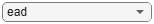"ead"; [DiscMeasure1,DiscData1] = modelDiscrimination(eadModel,EADData(TestInd,:),'ModelLevel',ModelLevel); modelDiscriminationPlot(eadModel,EADData(TestInd, :),'ModelLevel',ModelLevel,'SegmentBy','Marriage');```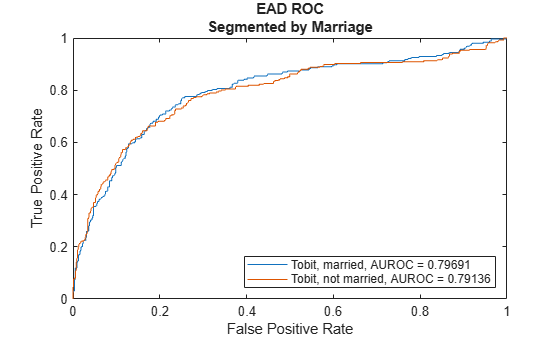Use `modelAccuracy` and then `modelAccuracyPlot` to show a scatter plot of the predictions.

```YData =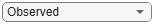"Observed"; [AccMeasure1,AccData1] = modelAccuracy(eadModel,EADData(TestInd,:),'ModelLevel',ModelLevel); modelAccuracyPlot(eadModel,EADData(TestInd,:),'ModelLevel',ModelLevel,'YData',YData);```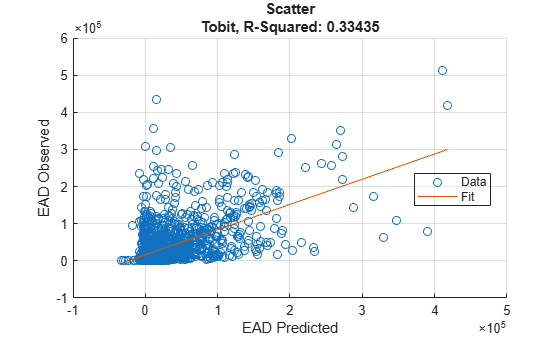## Input Arguments

collapse all

Exposure at default model, specified as a previously created `Regression` or `Tobit` object using `fitEADModel`.

Data Types: `object`

Data, specified as a `NumRows`-by-`NumCols` table with predictor and response values. The variable names and data types must be consistent with the underlying model.

Data Types: `table`

(Optional) Valid axis object, specified as an `ax` object that is created using `axes`. The plot will be created in the axes specified by the optional `ax` argument instead of in the current axes (gca). The optional argument `ax` must precede any of the input argument combinations.

Data Types: `object`

### Name-Value Arguments

Specify optional comma-separated pairs of `Name,Value` arguments. `Name` is the argument name and `Value` is the corresponding value. `Name` must appear inside quotes. You can specify several name and value pair arguments in any order as `Name1,Value1,...,NameN,ValueN`.

Example: `modelAccuracyPlot(eadModel,data(TestInd,:),'DataID','Testing','YData','residuals','XData','LTV')`

Data set identifier, specified as the comma-separated pair consisting of `'DataID'` and a character vector or string. The `DataID` is included in the output for reporting purposes.

Data Types: `char` | `string`

Model level, specified as the comma-separated pair consisting of `'ModelLevel'` and a character vector or string.

Note

`Regression` models support all three model levels, but a `Tobit` model supports model levels only for `'ead'` and `'conversionMeasure'`.

Data Types: `char` | `string`

EAD values predicted for `data` by the reference model, specified as the comma-separated pair consisting of `'ReferenceEAD'` and a `NumRows`-by-`1` numeric vector. The scatter plot output is plotted for both the `eadModel` object and the reference model.

Data Types: `double`

Identifier for the reference model, specified as the comma-separated pair consisting of `'ReferenceID'` and a character vector or string. `'ReferenceID'` is used in the scatter plot output for reporting purposes.

Data Types: `char` | `string`

Data to plot on x-axis, specified as the comma-separated pair consisting of `'XData'` and a character vector or string for one of the following:

• `'predicted'` — Plot the predicted EAD values in the x-axis.

• `'observed'` — Plot the observed EAD values in the x-axis.

• `'residuals'` — Plot the residuals in the x-axis.

• VariableName — Use the name of the variable in the `data` input, not necessarily a model variable, to plot in the x-axis.

Data Types: `char` | `string`

Data to plot on y-axis, specified as the comma-separated pair consisting of `'YData'` and a character vector or string for one of the following:

• `'predicted'` — Plot the predicted EAD values in the y-axis.

• `'observed'` — Plot the observed EAD values in the y-axis.

• `'residuals'` — Plot the residuals in the y-axis.

Data Types: `char` | `string`

## Output Arguments

collapse all

Figure handle for the scatter and line objects, returned as handle object.

collapse all

### Model Accuracy Plot

The `modelAccuracyPlot` function returns a scatter plot of observed vs. predicted loss given default (EAD) data with a linear fit and reports the R-square of the linear fit.

The `XData` name-value pair argument allows you to change the x values on the plot. By default, predicted EAD values are plotted in the x-axis, but predicted EAD values, residuals, or any variable in the `data` input, not necessarily a model variable, can be used as x values. If the selected `XData` is a categorical variable, a swarm chart is used. For more information, see `swarmchart`.

The `YData` name-value pair argument allows users to change the y values on the plot. By default, observed EAD values are plotted in the y-axis, but predicted EAD values or residuals can also be used as y values. `YData` does not support table variables.

The linear fit and reported R-squared value always correspond to the linear regression model with the plotted y values as response and the plotted x values as the only predictor.

 Baesens, Bart, Daniel Roesch, and Harald Scheule. Credit Risk Analytics: Measurement Techniques, Applications, and Examples in SAS. Wiley, 2016.

 Bellini, Tiziano. IFRS 9 and CECL Credit Risk Modelling and Validation: A Practical Guide with Examples Worked in R and SAS. San Diego, CA: Elsevier, 2019.

 Brown, Iain. Developing Credit Risk Models Using SAS Enterprise Miner and SAS/STAT: Theory and Applications. SAS Institute, 2014.

 Roesch, Daniel and Harald Scheule. Deep Credit Risk. Independently published, 2020.